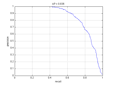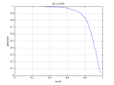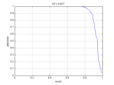Project 4 (Face detection with a sliding window)

- Emanuel Zgraggen (ez), November 9th, 2011

Introduction

The task given by this assignment is to implement a sliding window face detector based on concepts presented in Dalal-Triggs 2005 and Viola-Jones 2001. The algorithm will be evaluated using a common benchmark for face detection (CMU+MIT test set).

Approach

The sliding window concept is quite simple. By looping over an image with a constant step size, small image patches (36 x 36 pixels) are extracted at different scales. For each patch, the algorithm makes a decision if it contains a face or not. The focus of this project lies on the following 3 steps. They are explained in more detail further below.

• Find a good feature representation for image patches.
• Collect positive and negative training data.
• Train a classifier using collected data.

Feature representation
As presented in Dalal-Triggs 2005 this solution uses Histograms of Oriented Gradient (HOG) to represent an image patch. The algorithm uses this specific implementation by Pedro Felzenszwalb. Some experiments showed that a bin size of 5 (800-dimensional feature vector for each image patch) seems to be a good trade-off between accuracy and speed.

Collect training data
The algorithm uses an iterative strategy to collect hard negative image patches. The classifier gets first trained on randomly sampled non-face patches. In the next iteration the algorithm runs the classifier on scenes which have no faces and every detection is a false positive. A new classifier gets then retrained with the initial random samples and the mined false positive patches.

Positive training data / Random negative samples and two iterations of hard negatives.Train a classifier
Two different types of classifiers have been evaluated in this project (see results section for comparison), linear and non-linear (radial basis function, RBF) SVMs. When training a linear SVM the lambda parameter has an influence on the accuracy. Some experiments showed that lambda = 1.0 is good value. For the RBF classifier a sigma value of 2.0 is used.

Average precision of a linear SVM with different lambda values.

Non-linear classifiers tend to be more accurate in general, the problem is that they are slower to train and run. Viola-Jones 2001 introduced the concept of a cascade architecture. They key idea is to chain simpler, smaller classifiers to obtain a complex classifier that is fast in rejecting "easy" non-faces. This solution trains such cascades by combining small (400 positive, 400 negative examples) classifiers. The negative training data for each level is obtained by using the false positive patches of the higher up classifier.

Results

Approach Average Precision Precision Recall Comments
Linear SVM 0.836Lambda = 1
Detection time = 1466 sec
Positive training samples = 2000
Negative training samples = 3444
Hard mining iterations = 0
Linear SVM 0.870Lambda = 1
Detection time = 1487 sec
Positive training samples = 2000
Negative training samples = 3594
Hard mining iterations = 2
Linear SVM 0.890Lambda = 1
Detection time = 5646 sec
Positive training samples = 6000
Negative training samples = 88282
Hard mining iterations = 0
Non-Linear SVM (RBF) 0.927Lambda = 1
Sigma = 2.0
Detection time = 4646 sec
Positive training samples = 2000
Negative training samples = 2769
Hard mining iterations = 2
Non-Linear SVM (RBF) Cascade 0.831Lambda = 1
Sigma = 2.0
Detection time = 1557 sec
Positive training samples per level = 400
Negative training samples per level = 400
Number of levels = 6

Some example results.

Discussion

The non-linear classifiers get better average precisions, but are slower at detection time. Using a cascade architecture helps to make it faster, but average precisions also drops. For mining hard negatives, I had the problem that the initial classifier is usually already pretty good, so that after a few iterations there were almost no false positives anymore. I added a lot of additional non-face scenes to deal with that issue. In general, adding hard negative data helps to increase the average precision (see the first two entries in the result table). Using drastically more negative training samples helps as well (third entry).